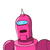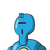# Fill in the blanks.i. The sum of three angles of a triangle isii. Two circles are congruent if theirare equal. January 4, 2022 by Ariana

Fill in the blanks.
i. The sum of three angles of a triangle is
ii. Two circles are congruent if their
are equal.
iii. The
is the greatest side in a right triangle.
iv. The exterior angle of a triangle is equal to the sum of its
angles.
If there are 9 values in a data set arranged in descending order, then
the median is the
term.
V.​

### 2 thoughts on “Fill in the blanks.<br />i. The sum of three angles of a triangle is<br />ii. Two circles are congruent if their<br />are equal.<b”

1.1) 180

3) hypotenuse

2.